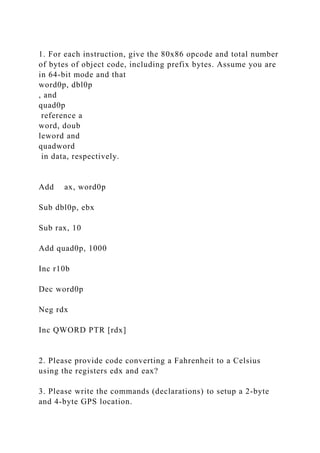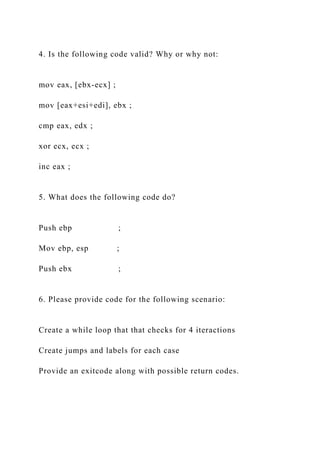Seu SlideShare está sendo baixado. ×

# 1. For each instruction, give the 80x86 opcode and total number of b.docx

Anúncio
Anúncio
Anúncio
Anúncio
Anúncio
Anúncio
Anúncio
Anúncio
Anúncio
Anúncio
AnúncioCarregando em…3
×

1 de 3 Anúncio

# 1. For each instruction, give the 80x86 opcode and total number of b.docx

1. For each instruction, give the 80x86 opcode and total number of bytes of object code, including prefix bytes. Assume you are in 64-bit mode and that
word0p, dbl0p
, and
reference a
word, doub
leword and
in data, respectively.

Sub dbl0p, ebx

Sub rax, 10

Inc r10b

Dec word0p

Neg rdx

Inc QWORD PTR [rdx]

2. Please provide code converting a Fahrenheit to a Celsius using the registers edx and eax?

3. Please write the commands (declarations) to setup a 2-byte and 4-byte GPS location.

4. Is the following code valid? Why or why not:

mov eax, [ebx-ecx] ;

mov [eax+esi+edi], ebx ;

cmp eax, edx ;

xor ecx, ecx ;

inc eax ;

5. What does the following code do?

Push ebp                  ;

Mov ebp, esp           ;

Push ebx                  ;

6. Please provide code for the following scenario:

Create a while loop that that checks for 4 iteractions

Create jumps and labels for each case

Provide an exitcode along with possible return codes.
.

1. For each instruction, give the 80x86 opcode and total number of bytes of object code, including prefix bytes. Assume you are in 64-bit mode and that
word0p, dbl0p
, and
reference a
word, doub
leword and
in data, respectively.

Sub dbl0p, ebx

Sub rax, 10

Inc r10b

Dec word0p

Neg rdx

Inc QWORD PTR [rdx]

2. Please provide code converting a Fahrenheit to a Celsius using the registers edx and eax?

3. Please write the commands (declarations) to setup a 2-byte and 4-byte GPS location.

4. Is the following code valid? Why or why not:

mov eax, [ebx-ecx] ;

mov [eax+esi+edi], ebx ;

cmp eax, edx ;

xor ecx, ecx ;

inc eax ;

5. What does the following code do?

Push ebp                  ;

Mov ebp, esp           ;

Push ebx                  ;

6. Please provide code for the following scenario:

Create a while loop that that checks for 4 iteractions

Create jumps and labels for each case

Provide an exitcode along with possible return codes.
.

Anúncio
Anúncio

### 1. For each instruction, give the 80x86 opcode and total number of b.docx

1. 1. 1. For each instruction, give the 80x86 opcode and total number of bytes of object code, including prefix bytes. Assume you are in 64-bit mode and that word0p, dbl0p , and quad0p reference a word, doub leword and quadword in data, respectively. Add ax, word0p Sub dbl0p, ebx Sub rax, 10 Add quad0p, 1000 Inc r10b Dec word0p Neg rdx Inc QWORD PTR [rdx] 2. Please provide code converting a Fahrenheit to a Celsius using the registers edx and eax? 3. Please write the commands (declarations) to setup a 2-byte and 4-byte GPS location.
2. 2. 4. Is the following code valid? Why or why not: mov eax, [ebx-ecx] ; mov [eax+esi+edi], ebx ; cmp eax, edx ; xor ecx, ecx ; inc eax ; 5. What does the following code do? Push ebp ; Mov ebp, esp ; Push ebx ; 6. Please provide code for the following scenario: Create a while loop that that checks for 4 iteractions Create jumps and labels for each case Provide an exitcode along with possible return codes.